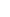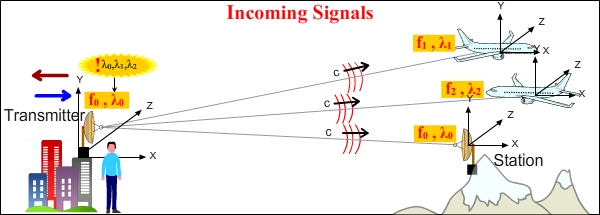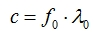17. ELECTROMAGNETIC WAVE SPEEDThere is the following equation among a waves speed, frequency, and wavelength.Wave Speed = Wave Frequency x WavelengthThis general wave speed equation applies for electromagnetic waves, as well. It has been presented as c = f . λ in physics up until now and it has been accepted to be unchangeable (c: the speed of light, f: wave frequency, λ: wavelength). c = f . λ equation is correct; however, it is not valid for all conditions. In the part we obtained (c+v) (c-v) mathematics for The Electromagnetic Theory, we saw that INCOMING and OUTGOING signals needed to be evaluated separately. Therefore, Wave Speed equation had to be rewritten according to the rules of (c+v) (c-v) mathematics.17.1. WAVE SPEED EQUATION FOR INCOMING SIGNALSWe saw before that we can assume that, of the frames that are in motion relative to each other, one is motionless and the other is in motion. The figure below was drawn relative to the reference systems that receive the signal (the planes and the station on the mountain).  - The transmitter emits signals at f0 frequency over λ0 wavelength relative to its own reference system.  - Relative to the reference system of the plane at the top, the transmitter moves away from itself in the direction of the red arrow.  - Relative to the reference system of the plane at the bottom, the transmitter approaches itself in the direction of the blue arrow.In the figure, the speeds of the signals are shown relative to their targets.We have seen before that the plane at the top receives the signal at f1 frequency and over λ1 wavelength. We also saw that, for a reference system, the incoming signal speed which is coming to the reference system itself is always c. Therefore, Wave speed equation for the plane at the top is as follows: c = f1 . λ1The plane at the bottom receives the signal at f2 frequency and over λ2 wavelength. incoming signal speed relative to its own reference system is c. The signal speed equation for the plane at the bottom should be as follows: c = f2 . λ2The station is motionless relative to the receiver. Therefore, it receives the signal at the same f0 frequency and over the same λ0 wavelength as the transmitter. Incoming signal speed relative to its own reference system is c. From this, we can write the equation: c = f0 . λ017.2. WAVE SPEED EQUATION FOR OUTGOING SIGNALSThe figure below was drawn relative to the reference system of the transmitter. We saw that the signals go to the plane moving away at c+v speed, the plane approaching at c-v speed, and to the station on the mountain at c speed relative to the reference system of the transmitter. On the other hand, we also saw that, although the transmitter was manufactured in a way that it emits signals at f0 frequency and over λ0 wavelength, if a signal it emits goes to an object in motion, the signal wavelength changes at the time of signal emission.The change in the wavelength of a signal which is sent is obvious only and only on the side that receives the signal. The man who stands next to the transmitter cannot feel or measure the signals that set out from the transmitter and go in the direction of the planes over different wavelengths.In the figure, the signal speeds are shown relative to the transmitter of the signal.We saw that, even though the frequency of the transmitter doesnt change, the wavelengths of the signals going to the planes change. By using this information in Wave Speed equation, we can find the speeds of the signals going to the planes relative to the reference system of the transmitter.Therefore, the general rule for a signal in motion is as follows: The speed of a signal going to an object that is in motion is equal to the multiplication of the signal frequency of the reference system that emits the signal and the signal wavelength measured at the reference system receiving the signal. Again, Id like to emphasize that this signal speed value is relative to the reference system that sends the signal. We can briefly show this equation as follows: Outgoing Signal Speed = Frequency of Transmitter x Wavelength at Receiver = c ± vLets go back to the example we used on the topic The 44th Bit in the part about Byte Shift. We calculated wavelength changes for signals that go to planes that approach and move away and also signal speeds before. Lets calculate signal speeds with the help of wave speed equation this time. We see the calculated signal speeds in the table below. As can be seen, same values are obtained for signal speeds.This connection between wave speed, frequency and wavelength can be clearly seen in (c+v) (c-v) mathematics already. (*)Factory setting of the signal sourceWavelength changeWe obtain the following third equation from equation number 1 and 2.Wave Speed Equation(c±v) : Outgoing signal speed, f0 : Frequency of transmitter, λ1 : Signal wavelength measured at the receiverThis equation gives us the speed rule that the signal that is emitted from a transmitter is subject to. We already know that the part c±v in the equation is the OUTGOING signal speed relative to the reference system of the transmitter.(*) Im kidding. Equation number 1 and 2 had been right in front of my eyes for years and I couldnt notice the equation number 3. I could have never obtained the third equation; I know that. I feel so lucky to have noticed it. As I said  Physics is really difficult.